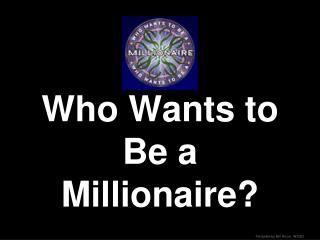# Who Wants to Be a Millionaire? - PowerPoint PPT PresentationDownload PresentationWho Wants to Be a Millionaire?

Who Wants to Be a Millionaire?
Download Presentation## Who Wants to Be a Millionaire?

- - - - - - - - - - - - - - - - - - - - - - - - - - - E N D - - - - - - - - - - - - - - - - - - - - - - - - - - -
##### Presentation Transcript

1. Who Wants to Be a Millionaire?

2. Click the appropriate dollar amount at right to proceed to the question _________________________ _____________________ _____________________ _____________________ ___________________ ___________________ __________________ _________________ _________________ _________________ _________________ ______________ ______________ ______________ ______________ ALGEBRA

5. 50/50 Fifty - Fifty • You’ve asked that two of the wrong answers be eliminated, leaving you with one wrong answer plus the correct one. Return to the Question

6. Correct Correct! Proceed to Next Question

8. Which statement is false? 50/50 The order in which 2 whole numbers are added does not affect the sum. The order in which two whole numbers are subtracted does not affect the difference A B The order in which two rational numbers are added does not affect the sum The order in which two rational numbers are multiplied does not affect the product. C D

9. Is the equation 3(2x-4) = -18 equivalent to 6x-12 = -18 ? 50/50 No, the equations are not equivalent. Yes, the equations are equivalent by the Associative Property of Multiplication. A B Yes, the equations are equivalent by the Communative Property of Multiplication. Yes, the equations are equivalent by the Distributive Property of Multiplication over Addition. C D

10. Identify the first incorrect step.Solve: 3(x +5) =2x +35Step 1: 3x +15 =2x +35Step 2: 5x +15 =35Step 3: 5x =20Step 4: x =4 50/50 Step 3 Step 1 A B Step 2 Step 4 C D

11. Which equation is equivalent to [ 7x-4 (x-3) ] + 1 = 16 ? 50/50 17x – 2 = 16 9x – 2 =16 A B 17x +13 = 16 9x + 37 = 16 C D

12. What is the solution for this equation? |2x − 3|= 5 50/50 x = -1 or x= 4 x = -4 or x= 4 A B x = -1 or x= 3 x = -4 or x = 3 C D

13. What is the multiplicative inverse of 1/2 50/50 -1/2 -2 A B 1/2 2 C D

14. Which expression is equivalent to x6 x2 ? 50/50 x7x3 x4x3 A B x5x3 x9x3 C D

15. The lengths of the sides of a triangle are y, y +1, and 7 centimeters. If the perimeter is 56 centimeters, what is the value of y? 50/50 24 25 A B 31 32 C D

16. Beth is two years older than Julio. Gerald is twice as old as Beth. Debra is twice as old as Gerald. The sum of their ages is 38. How old is Beth? 50/50 3 6 A B 8 5 C D

17. What is the x-intercept of the line defined by −2x +3y=12? 50/50 - 4 - 6 A B 4 6 C D

18. What is the equation of the line that has a slope of 4 and passes through the point(3,-10) ? 50/50 Y=4x -22 Y=4x + 22 A B Y=4x - 43 Y=4x + 43 C D

19. Which equation represents a line that is parallel toy =−5/4 x +2? 50/50 Y= -5/4x + 2 Y= 5/4x + 4 A B Y= 4/5x + 3 Y= -5/4x +1 C D

20. At what point do the lines represented by the equations 2x + y +1= 0 and 4x + y – 3= 0intersect? 50/50 (-1, 1) (2, -5) A B (2, 5) (1, -1) C D

21. Which is the factored form of 3a2 −24ab + 48b2? 50/50 3(a – 4b)(a – 4b) (3a – 8b)(a – 6b) A B (3a – 16b)(a – 3b) 3(a – 8b)(a – 8b) C D

22. Which of the following expressions is equal to (x + 2 ) + (x− 2 )(2x +1) ? 50/50 2x2 + x 2x2 – 2x A B 2x2 – 4x 4x2 + 2x C D